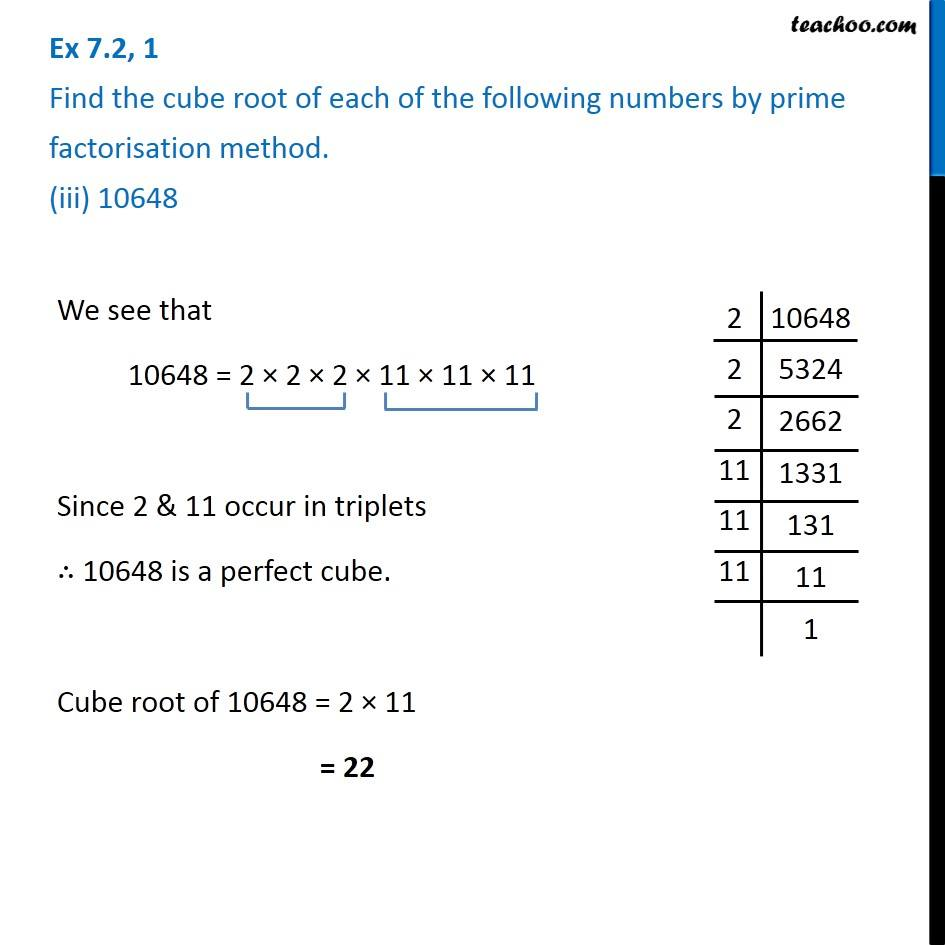Ex 7.2

Chapter 7 Class 8 Cubes and Cube Roots
Serial order wise### Transcript

Ex 7.2, 1 Find the cube root of each of the following numbers by prime factorisation method. (iii) 10648We see that 10648 = 2 × 2 × 2 × 11 × 11 × 11 Since 2 & 11 occur in triplets ∴ 10648 is a perfect cube. Cube root of 10648 = 2 × 11 = 22